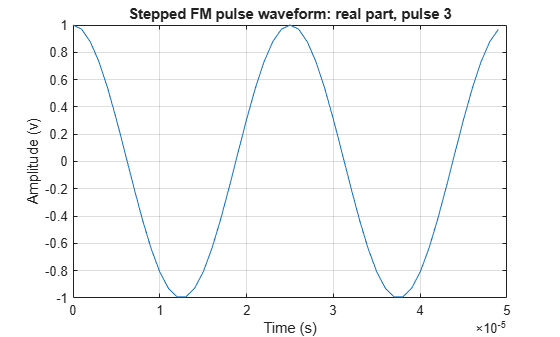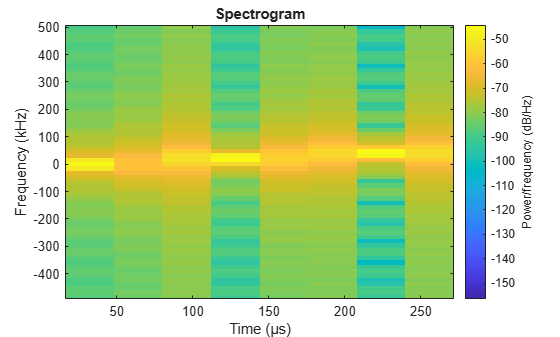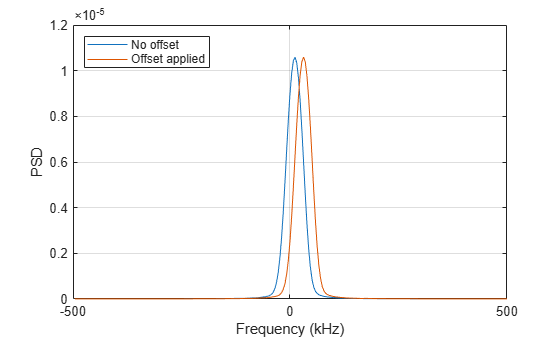Main Content

# phased.SteppedFMWaveform

Stepped FM pulse waveform

## Description

The SteppedFMWaveform object creates a stepped FM pulse waveform.

To obtain waveform samples:

1. Define and set up your stepped FM pulse waveform. See Construction.

2. Call step to generate the stepped FM pulse waveform samples according to the properties of phased.SteppedFMWaveform. The behavior of step is specific to each object in the toolbox.

Note

Starting in R2016b, instead of using the step method to perform the operation defined by the System object™, you can call the object with arguments, as if it were a function. For example, y = step(obj,x) and y = obj(x) perform equivalent operations. When the only argument to the step method is the System object itself, replace y = step(obj) by y = obj().

## Construction

sSFM = phased.SteppedFMWaveform creates a stepped FM pulse waveform System object, sSFM. The object generates samples of a linearly stepped FM pulse waveform.

sSFM = phased.SteppedFMWaveform(Name,Value) creates a stepped FM pulse waveform object, sSFM, with each specified property Name set to the specified Value. You can specify additional name-value pair arguments in any order as (Name1,Value1,...,NameN,ValueN).

## Properties

SampleRate

Sample rate

Signal sample rate, specified as a positive scalar. Units are Hertz. The ratio of sample rate to pulse repetition frequency (PRF) must be a positive integer — each pulse must contain an integer number of samples.

Default: 1e6

DurationSpecification

Method to set pulse duration

Method to set pulse duration (pulse width), specified as 'Pulse width' or 'Duty cycle'. This property determines how you set the pulse duration. When you set this property to 'Pulse width', then you set the pulse duration directly using the PulseWidth property. When you set this property to 'Duty cycle', you set the pulse duration from the values of the PRF and DutyCycle properties. The pulse width is equal to the duty cycle divided by the PRF.

Default: 'Pulse width'

PulseWidth

Pulse width

Specify the length of each pulse (in seconds) as a positive scalar. The value must satisfy PulseWidth <= 1./PRF.

Default: 50e-6

DutyCycle

Waveform duty cycle

Waveform duty cycle, specified as a scalar from 0 through 1, inclusive. This property applies when you set the DurationSpecification property to 'Duty cycle'. The pulse width is the value of the DutyCycle property divided by the value of the PRF property.

Default: 0.5

PRF

Pulse repetition frequency

Pulse repetition frequency, PRF, specified as a scalar or a row vector. Units are in Hz. The pulse repetition interval, PRI, is the inverse of the pulse repetition frequency, PRF. ThePRF must satisfy these restrictions:

• The product of PRF and PulseWidth must be less than or equal to one. This condition expresses the requirement that the pulse width is less than one pulse repetition interval. For the phase-coded waveform, the pulse width is the product of the chip width and number of chips.

• The ratio of sample rate to any element of PRF must be an integer. This condition expresses the requirement that the number of samples in one pulse repetition interval is an integer.

You can select the value of PRF using property settings alone or using property settings in conjunction with the prfidx input argument of the step method.

• When PRFSelectionInputPort is false, you set the PRF using properties only. You can

• implement a constant PRF by specifying PRF as a positive real-valued scalar.

• implement a staggered PRF by specifying PRF as a row vector with positive real-valued entries. Then, each call to the step method uses successive elements of this vector for the PRF. If the last element of the vector is reached, the process continues cyclically with the first element of the vector.

• When PRFSelectionInputPort is true, you can implement a selectable PRF by specifying PRF as a row vector with positive real-valued entries. But this time, when you execute the step method, select a PRF by passing an argument specifying an index into the PRF vector.

In all cases, the number of output samples is fixed when you set the OutputFormat property to 'Samples'. When you use a varying PRF and set the OutputFormat property to 'Pulses', the number of samples can vary.

Default: 10e3

PRFSelectionInputPort

Enable PRF selection input

Enable the PRF selection input, specified as true or false. When you set this property to false, the step method uses the values set in the PRF property. When you set this property to true, you pass an index argument into the step method to select a value from the PRF vector.

Default: false

FrequencyStep

Linear frequency step size

Specify the linear frequency step size (in hertz) as a positive scalar. The default value of this property corresponds to 20 kHz.

Default: 20e3

NumSteps

Specify the number of frequency steps as a positive integer. When NumSteps is 1, the stepped FM waveform reduces to a rectangular waveform.

Default: 5

FrequencyOffsetSource

Source of frequency offset

Source of frequency offset for the waveform, specified as 'Property' or 'Input port'.

• When you set this property to 'Property', the offset is determined by the value of the FrequencyOffset property.

• When you set this property to 'Input port', the FrequencyOffset is determined by the freqoffset input argument.

Default: 'Property'

FrequencyOffset

Frequency offset

Frequency offset in Hz, specified as a scalar.

#### Dependencies

This property applies when you set the FrequencyOffsetSource property to 'Input port'.

Default: 0 Hz

OutputFormat

Output signal format

Specify the format of the output signal as 'Pulses' or 'Samples'. When you set the OutputFormat property to 'Pulses', the output of the step method takes the form of multiple pulses specified by the value of the NumPulses property. The number of samples per pulse can vary if you change the pulse repetition frequency during the simulation.

When you set the OutputFormat property to 'Samples', the output of the step method is in the form of multiple samples. In this case, the number of output signal samples is the value of the NumSamples property and is fixed.

Default: 'Pulses'

NumSamples

Number of samples in output

Specify the number of samples in the output of the step method as a positive integer. This property applies only when you set the OutputFormat property to 'Samples'.

Default: 100

NumPulses

Number of pulses in output

Specify the number of pulses in the output of the step method as a positive integer. This property applies only when you set the OutputFormat property to 'Pulses'.

Default: 1

PRFOutputPort

Set this property to true to output the PRF for the current pulse using a step method argument.

#### Dependencies

This property can be used only when the OutputFormat property is set to 'Pulses'.

Default: false

CoefficientsOutputPort

Enable matched filter coefficients output port

Enable the matched filter coefficients output port, specified as false or true. When you set this property to false, the object does not provide the matched filter coefficients used during the simulation as an output. When you set this property to true, the object provides the matched filter coefficients used during the simulation as an output.

Default: false

## Methods

 bandwidth Bandwidth of stepped FM pulse waveform getMatchedFilter Matched filter coefficients for waveform plot Plot stepped FM pulse waveform reset Reset state of stepped FM pulse waveform object step Samples of stepped FM pulse waveform
Common to All System Objects
release

Allow System object property value changes

## Examples

collapse all

Create a stepped frequency pulse waveform object. Assume the default value, 1 MHz, for the sample rate. Then, plot the waveform.

Create the SteppedFMWaveform System object™ with 20 kHz frequency step size.

sSFM = phased.SteppedFMWaveform('NumSteps',3,'FrequencyStep',20e3); fs = sSFM.SampleRate;

Plot the third pulse of the wave using the phased.SteppedFMWaveform.plot method. Pass in the pulse number using the 'PulseIdx' name-value pair.

plot(sSFM,'PulseIdx',3);Alternatively, call the step method three times to obtain three pulses. Collect the three pulses in a single time series. Then plot the waveform using the plot function. You can see the full duty cycles of the pulses.

wavfull = []; wav = step(sSFM); wavfull = [wavfull;wav]; wav = step(sSFM); wavfull = [wavfull;wav]; wav = step(sSFM); wavfull = [wavfull;wav]; nsamps = size(wavfull,1); t = [0:(nsamps-1)]/fs*1e6; plot(t,real(wavfull)) xlabel('Time (\mu sec)') ylabel('Amplitude') gridPlot the spectrum using the spectrogram function. Assume an fft of 64 samples and a 50% overlap. Window the signal with a hamming function.

nfft1 = 64; nov = floor(0.5*nfft1); spectrogram(wavfull,hamming(nfft1),nov,nfft1,fs,'centered','yaxis')Apply a frequency offset to a stepped FM (SFM) pulse waveform. Plot the frequency spectrum of the waveform with and without a frequency offset applied.

Create a SFM waveform object which is configured to set the frequency offset from an input when the object is executed.

fs = 1e6; sSFM = phased.SteppedFMWaveform('SampleRate',fs,'NumSteps',2, ... 'FrequencyStep',20e3,'NumPulses',2,'FrequencyOffsetSource','Input port');

Execute the object two times. First set the frequency offset set to 0 Hz, and then to 2e4 Hz.

sfmwav = sSFM(0); sfmwav_foffset = sSFM(2e4);

Plot the frequency spectrum of the complex signals. The frequency offset signal is shifted to the right.

[Pxx,f] = pwelch(sfmwav,[],[],[],fs,'centered'); [Pxx_offset,foffset] = pwelch(sfmwav_foffset,[],[],[],fs,'centered'); plot(f/1000,Pxx,foffset/1000,Pxx_offset) ylabel('PSD'); xlabel('Frequency (kHz)'); legend({'No offset','Offset applied'},'Location','northwest'); grid on;expand all

## References

 Richards, M. A. Fundamentals of Radar Signal Processing. New York: McGraw-Hill, 2005.

## See Also

Introduced in R2011a

## Support

#### Exploring Hybrid Beamforming Architectures for 5G Systems

Download white paper